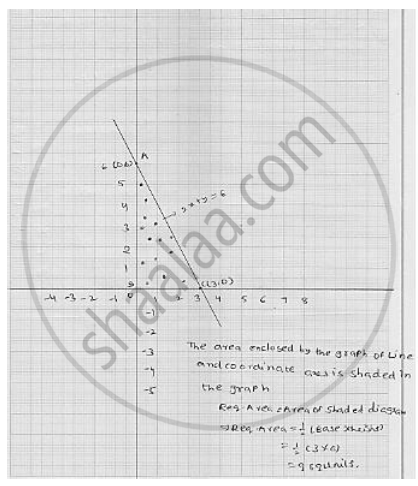# Draw the Graph of the Equation 2x + Y = 6. Shade the Region Bounded by the Graph and the Coordinate Axes. Also, Find the Area of the Shaded Region. - Mathematics

Draw the graph of the equation 2x + y = 6. Shade the region bounded by the graph and the
coordinate axes. Also, find the area of the shaded region.

#### Solution

We have

2x + y= 6

y = 6 - 2x     ................ (1)

Putting  x = 3 in (1) , we get y = 6 - 2 × 3 = 0

Putting  x = 0 in (1) , we get y = 6 - 2 × 0 = 6

Thus, we obtained the following table giving coordinates of two points on the line
represented by the equation

2x + y = 6

 x 3 0 y 0 6

The graph of line   2x + y = 6Concept: Graph of a Linear Equation in Two Variables
Is there an error in this question or solution?

#### APPEARS IN

RD Sharma Mathematics for Class 9
Chapter 7 Linear Equations in Two Variables
Exercise 7.3 | Q 13

Share